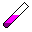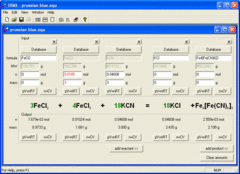Search
SoftwareEBAS is a program for balancing chemical equations and stoichiometry calculations. Built in reagent database and concentration and ideal gas calculators simplify solving of all kinds of stoichiometry questions, both textbook and real life ones. To solve most questions it is enough to enter known information - program immediately balances equation, calculates molar masses of all substances, finds out limiting reagents and calculates stoichiometric amounts of all compunds involved. Concentration calculator helps in conversions between volume, mass (or number of moles) and concentrations, while ideal gas calculator helps to convert between mass/number of moles and volume of the gas for any temperature and pressure values. Balanced equation can be exported through clipboard in numerous formats - TXT, RTF, HTML, UBBC or LaTeX.